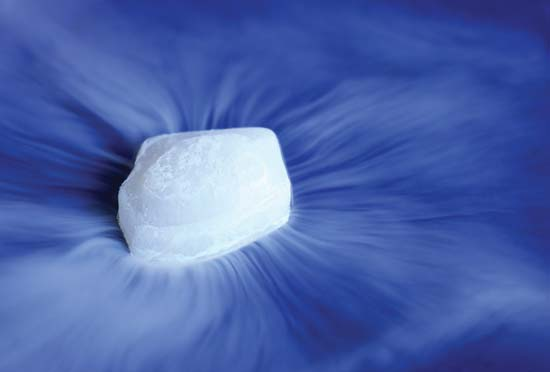# Thermodynamics in Dry ice

Chemistry Level 2Dry ice, sometimes referred to as "cardice" or as "card ice" (chiefly British English), is the solid form of carbon dioxide. It is used primarily as a cooling agent. Its advantages include lower temperature than that of water ice and not leaving any residue (other than incidental frost from moisture in the atmosphere). Dry ice sublimates at $-78.5$ °C ($-109.3$ °F) at Earth atmospheric pressures. Which of the following statements is correct about the sublimation of dry ice? ($\Delta H$, $\Delta S$ and $\Delta G$ mean the change in enthalpy, entropy and Gibbs free energy, respectively.)

a) $\Delta H > 0$ and $\Delta S > 0$, and $\Delta G < 0$ for all temperatures.

b) $\Delta H > 0$ and $\Delta S < 0$, and $\Delta G > 0$ for all temperatures.

c) $\Delta H < 0$ and $\Delta S > 0$, and $\Delta G < 0$ for all temperatures.

d) $\Delta H > 0$ and $\Delta S > 0$, and $\Delta G < 0$ for high temperatures.

×